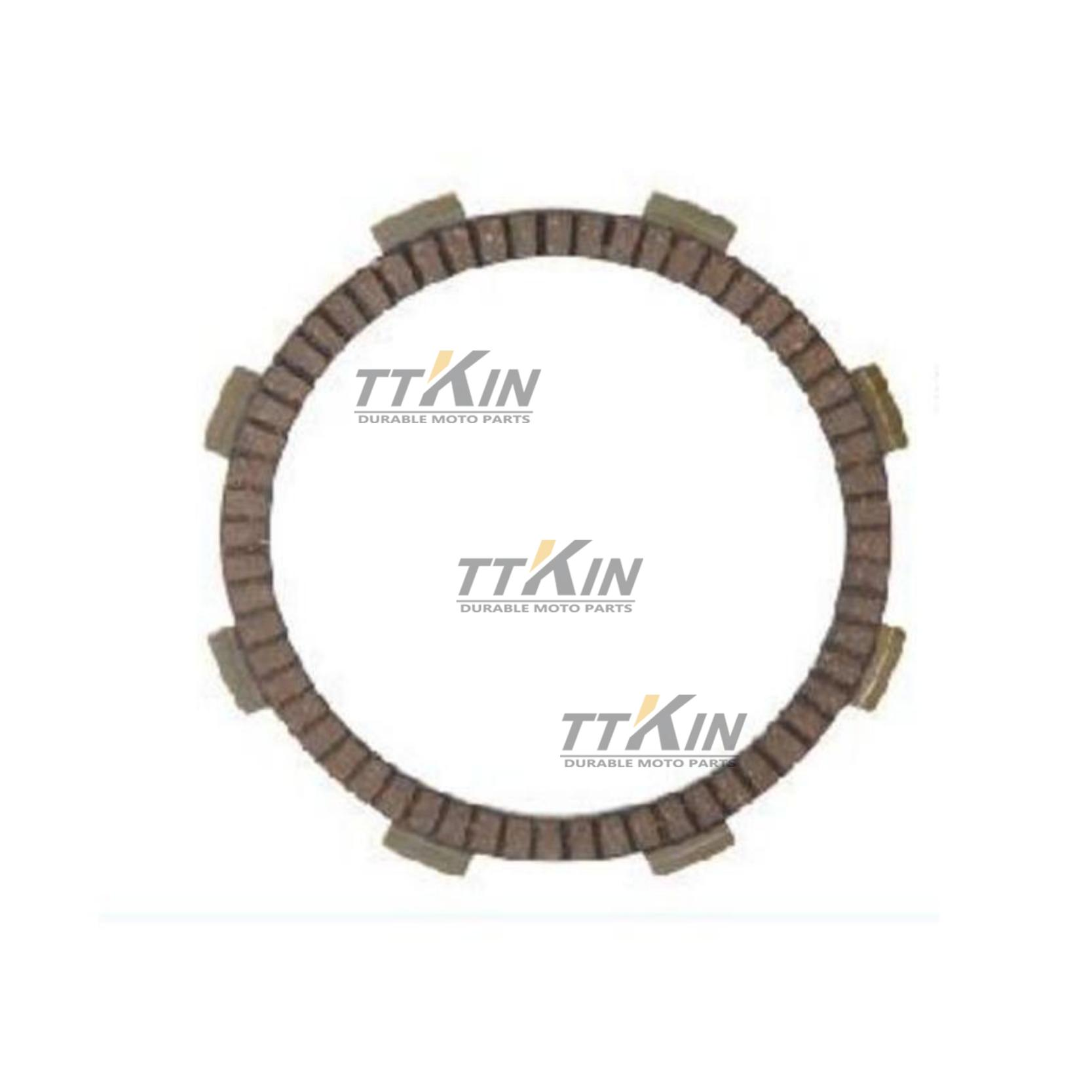# The difference and selection of motorcycle brake pads

08/09/2022
The difference and selection of motorcycle brake pads
Motorcycle brake pad is an important factor related to life safety, so you must not be careless in choosing it.
A. How to evaluate the quality of brake pads?
The quality of brake pads is not simply to choose the best ones for racing cars. We want to see:
1) What is the friction coefficient of the brake pad?
2) How wide is the effective working temperature of the brake pad?
3) How fast does the brake pad wear out?
4) How loud is the noise when braking?
5) How much force powder is released from the force pad during braking?
6) Is the friction provided by the brake pads linear? Easy to master?
The higher the friction coefficient, the higher the work efficiency of the brake pad. However, choosing the brake pads for your car does not mean that the higher the quality, the more suitable it is for your needs. The weight and speed of different vehicles are different. For vehicles with low speed and light body, the force skin with high friction coefficient will damage the handling and affect the driving experience.
B. What is the coefficient of friction?
The coefficient of friction (U) is a quantitative simplification of friction resistance. One of the simplest calculation methods is to divide the frictional resistance (FR) by the force (FN) required to counteract the frictional force and successfully pull the animal. The formula of friction coefficient is u = FR / FN.
Another measurement method is to use gravity, that is, the weight (W) of the object to measure: u = FR / W. For example, in the left figure, the weight of a book is w. divided by the force required to pull the book on the table, the weight measured by the slingshot pound is the coefficient of friction between the book and the table.
The friction coefficient is divided into at least four different versions based on the different conditions that constitute the friction resistance (FR) and the force that breaks the friction resistance. Among them, the common ones are static friction coefficient and dynamic friction coefficient. Of course, the dynamic friction coefficient is applied to the motorcycle braking system.